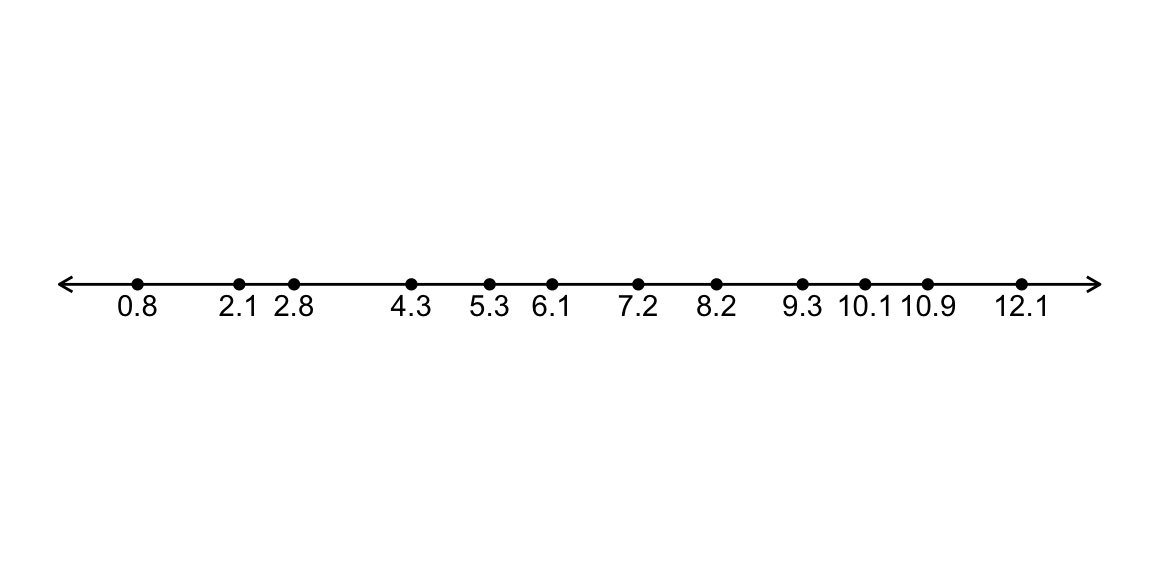# Sign test

## Data Setting

Population: $$Y \sim$$ something with c.d.f $$F_Y(y) = P(Y \le y)$$

Parameter: $$M = F^{-1}_Y(0.5)$$, the population median

Sample: $$n$$ i.i.d from population: $$Y_1, \ldots, Y_n$$

Null hypothesis: $$H_0: M = M_0$$

Consider the hypothesis $$H_0: M = M_0$$

Imagine transforming the $$Y_i, \, i = 1, \ldots, n$$ to $X_i = \begin{cases} 1, & Y_i \le M_0 \\ 0, & Y_i > M_0 \end{cases}$

If the null hypothesis is true $$M = M_0$$, what is $$P(X_i = 1)$$?

(You can assume $$Y$$ is a continuous distribution)

## Sign test

To test $$H_0: M = M_0$$, perform a Binomial test on $$X_i = \pmb{1}\{Y_i \le M_0 \}$$ with $$H_0: p = 0.5$$.

## Example

Consider a sample, $$n = 12$$, with the sample values:Consider testing $$H_0: M = 4$$ versus a two-sided alternative $$H_A: M \ne 4$$ (at the $$\alpha = 0.05$$ level).

$$X_i = \pmb{1}\{Y_i \le 4 \}$$

$$\hat{p}_{M_0} = \frac{1}{n}\sum_{i = 1}^{n}X_i = 0.25$$

$$Z(p_0 = 0.5) = \frac{\hat{p}_{M_0} - p_0}{\sqrt{p_0(1-p_0)/n}} = -1.73$$

Compare to $$z_{1-\alpha/2} = 1.96$$

We fail to reject the null hypothesis.

## Your turn: 95% Confidence Interval

We can invert the test by considering all $$M_0$$ for which we would fail to reject the null hypothesis $$H_0: M = M_0$$.

Would you reject for the value on your slip of paper?

Why do we only need to consider the actual sample values?

## Your turn: 95% Confidence Interval

##     m_0
## 1   0.8
## 2   2.1
## 3   2.8
## 4   4.3
## 5   5.3
## 6   6.1
## 7   7.2
## 8   8.2
## 9   9.3
## 10 10.1
## 11 10.9
## 12 12.1

95% confidence interval for $$M$$ is $$(\qquad, \qquad)$$

## Confidence interval in general

Solve for $$M_0$$ that satisfy (i.e. not in rejection region)

$\left| \frac{X/n - 0.5}{0.5 \sqrt{n}} \right| < z_{1-\alpha/2}, \quad \text{where } X = \text{number of observations } \le M_0$

$-z_{1-\alpha/2} < \frac{X/n - 0.5}{0.5 / \sqrt{n}} < z_{1-\alpha/2}$

\begin{aligned} n(0.5 -z_{1-\alpha/2}\frac{0.5}{\sqrt{n}}) &< X < n(0.5 + z_{1-\alpha/2}\frac{0.5}{\sqrt{n}}) \\ \frac{1}{2}(n -z_{1-\alpha/2}\sqrt{n}) &< X < \frac{1}{2}(n + z_{1-\alpha/2}\sqrt{n}) \end{aligned}

## Confidence interval in general

So, $$M_0$$ is in interval if the number of observations smaller than $$M_0$$ is between: $\frac{1}{2}(n -z_{1-\alpha/2}\sqrt{n}) \quad \text{ and } \quad \frac{1}{2}(n + z_{1-\alpha/2}\sqrt{n})$

The smallest value that satisifies this is the $\left(\frac{1}{2}(n -z_{1-\alpha/2}\sqrt{n})\right)^{\text{th}} \text{ smallest observation}$

The largest value that satisifies this is the $\left(\frac{1}{2}(n + z_{1-\alpha/2}\sqrt{n}) + 1\right)^{\text{th}} \text{ smallest observation}$

## Confidence interval for median

Approximate (based on approximate Binomial test) confidence interval for the median:

\begin{aligned} \Biggl( \left(\frac{n - z_{1-\alpha/2}\sqrt{n}}{2} \right)^{\text{th}} \text{ smallest observation}, \\ \left(\frac{n + z_{1-\alpha/2}\sqrt{n}}{2} + 1 \right)^{\text{th}} \text{ smallest observation}\Biggr) \end{aligned}

May need to round $$(.)^\text{th}$$ to nearest integers

## Example, continued

$$n = 12, \alpha = 0.05 \implies$$

\begin{aligned} &\Biggl(\left(\frac{12 - 1.96\sqrt{12}}{2} \right)^{\text{th}} \text{ smallest observation}, \\ &\qquad \left(\frac{12 +1.96\sqrt{12}}{2} + 1 \right)^{\text{th}} \text{ smallest observation} \Biggr) \\ &\left(\left(2.61\right)^{\text{th}} \text{ smallest observation}, \left(10.40\right)^{\text{th}} \text{ smallest observation} \right)\\ &\left(3^{\text{rd}} \text{ smallest observation}, 10^{\text{th}} \text{ smallest observation} \right) \\ &\left(2.8, 10.1 \right) \end{aligned}

## Sign test for discrete distributions/data

1. Remove all values exactly equal to $$M_0$$
2. Proceed with test as usual (with a reduced sample size $$n$$)

## Sign test: exactness

Finite sample exact? No

• Discrete nature of data means we can’t achieve a lot of signficance levels
• Normal approximation is only an approximation…

Assymptotically exact? Yes

## Sign test: consistency

The sign test test is consistent. Comes from Binomial test being consistent (which comes from Z-test being consistent).

Signed Rank test